Anúncio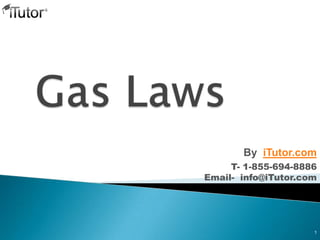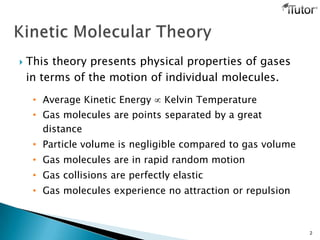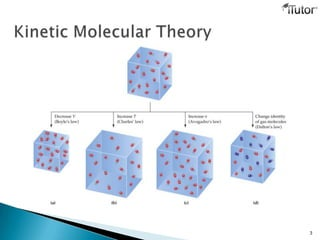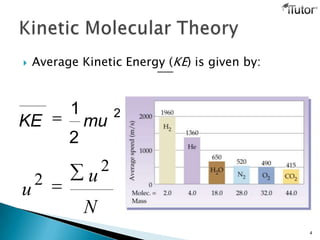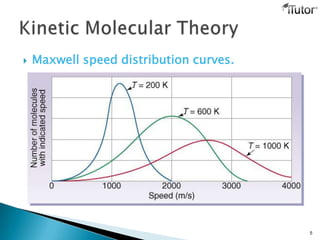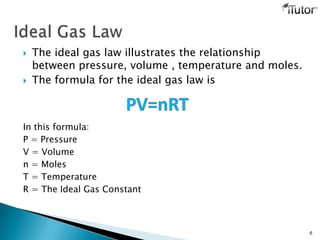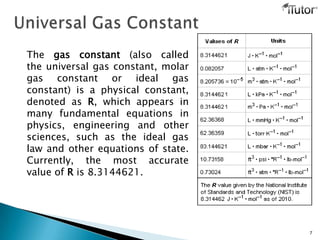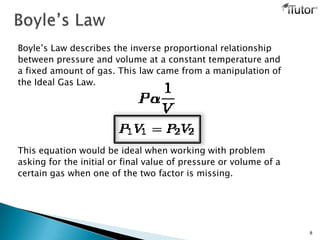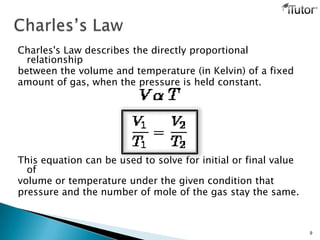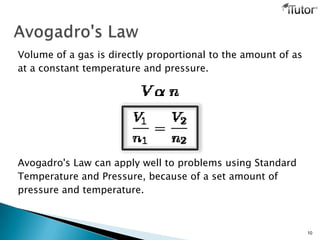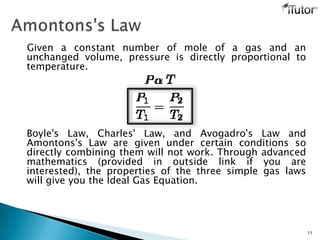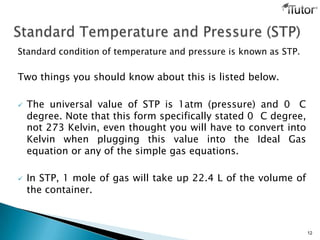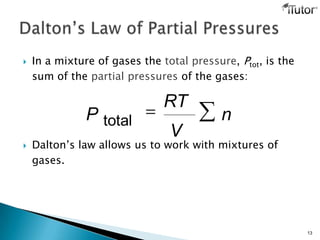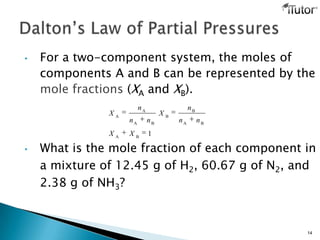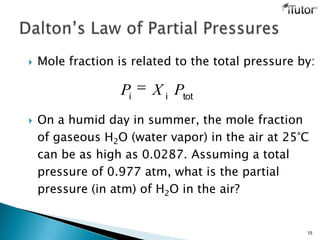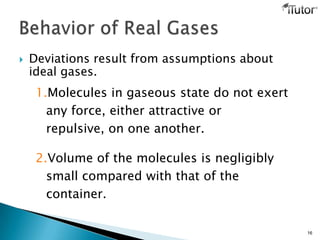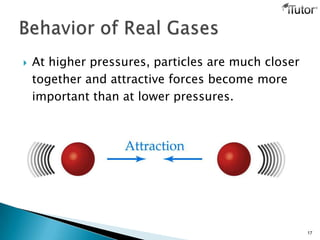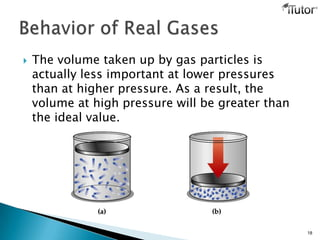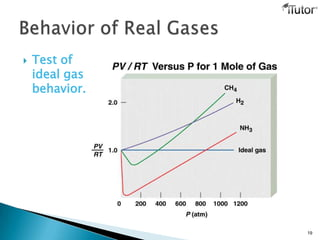1 de 20
Anúncio

### Gas Laws

1. 1 T- 1-855-694-8886 Email- info@iTutor.com By iTutor.com
2.  This theory presents physical properties of gases in terms of the motion of individual molecules. • Average Kinetic Energy Kelvin Temperature • Gas molecules are points separated by a great distance • Particle volume is negligible compared to gas volume • Gas molecules are in rapid random motion • Gas collisions are perfectly elastic • Gas molecules experience no attraction or repulsion 2
3. 3
4.  Average Kinetic Energy (KE) is given by: 4 KE 1 2 mu 2 u 2 u 2 N
5.  Maxwell speed distribution curves. 5
6.  The ideal gas law illustrates the relationship between pressure, volume , temperature and moles.  The formula for the ideal gas law is In this formula: P = Pressure V = Volume n = Moles T = Temperature R = The Ideal Gas Constant 6
7. The gas constant (also called the universal gas constant, molar gas constant or ideal gas constant) is a physical constant, denoted as R, which appears in many fundamental equations in physics, engineering and other sciences, such as the ideal gas law and other equations of state. Currently, the most accurate value of R is 8.3144621. 7
8. Boyle’s Law describes the inverse proportional relationship between pressure and volume at a constant temperature and a fixed amount of gas. This law came from a manipulation of the Ideal Gas Law. This equation would be ideal when working with problem asking for the initial or final value of pressure or volume of a certain gas when one of the two factor is missing. 8
9. Charles's Law describes the directly proportional relationship between the volume and temperature (in Kelvin) of a fixed amount of gas, when the pressure is held constant. This equation can be used to solve for initial or final value of volume or temperature under the given condition that pressure and the number of mole of the gas stay the same. 9
10. Volume of a gas is directly proportional to the amount of as at a constant temperature and pressure. Avogadro's Law can apply well to problems using Standard Temperature and Pressure, because of a set amount of pressure and temperature. 10
11. Given a constant number of mole of a gas and an unchanged volume, pressure is directly proportional to temperature. Boyle's Law, Charles' Law, and Avogadro's Law and Amontons's Law are given under certain conditions so directly combining them will not work. Through advanced mathematics (provided in outside link if you are interested), the properties of the three simple gas laws will give you the Ideal Gas Equation. 11
12. Standard condition of temperature and pressure is known as STP. Two things you should know about this is listed below.  The universal value of STP is 1atm (pressure) and 0 C degree. Note that this form specifically stated 0 C degree, not 273 Kelvin, even thought you will have to convert into Kelvin when plugging this value into the Ideal Gas equation or any of the simple gas equations.  In STP, 1 mole of gas will take up 22.4 L of the volume of the container. 12
13.  In a mixture of gases the total pressure, Ptot, is the sum of the partial pressures of the gases:  Dalton’s law allows us to work with mixtures of gases. 13 n V RT P total
14. 1BA BA B B BA A A XX nn n X nn n X 14 • For a two-component system, the moles of components A and B can be represented by the mole fractions (XA and XB). • What is the mole fraction of each component in a mixture of 12.45 g of H2, 60.67 g of N2, and 2.38 g of NH3?
15. totii PXP 15  Mole fraction is related to the total pressure by:  On a humid day in summer, the mole fraction of gaseous H2O (water vapor) in the air at 25°C can be as high as 0.0287. Assuming a total pressure of 0.977 atm, what is the partial pressure (in atm) of H2O in the air?
16.  Deviations result from assumptions about ideal gases. 1.Molecules in gaseous state do not exert any force, either attractive or repulsive, on one another. 2.Volume of the molecules is negligibly small compared with that of the container. 16
17.  At higher pressures, particles are much closer together and attractive forces become more important than at lower pressures. 17
18.  The volume taken up by gas particles is actually less important at lower pressures than at higher pressure. As a result, the volume at high pressure will be greater than the ideal value. 18
19.  Test of ideal gas behavior. 19